## NON-NEWTONIAN FLUIDS

A Newtonian Fluid is one where there is a linear relationship between stress and strain-rate: the ratio of stress to strain-rate is the viscosity of the fluid. A Hookean solid is one where there is a linear relationship between stress and strain: the ratio of stress to strain is the modulus of the solid. Many materials have intermediate properties between those of a Newtonian fluid and a Hookean solid. If the properties are predominantly solid-like, the materials are called non-Hookean and the materials are described as viscoelastic. If they are predominantly fluid-like, they are called non-Newtonian and the materials are described as elasticoviscous.
A non-Newtonian fluid has, therefore, a simultaneously elastic and viscous nature. In fact, all fluids are non-Newtonian on an appropriate time-scale, though for many common fluids such as air and water the time-scale is extremely short. When the time-scale of a flow tf is much less than the relaxation time tr of an elasticoviscous material, elastic effects dominate. This typically happens when there are abrupt changes in flow geometry. When on the other hand tf is much greater than tr elastic effects relax sufficiently for viscous effects to dominate. This typically happens when there are not abrupt changes in flow geometry. The ratio of tf to tr is a dimensionless number of particular significance in the study of flow of non-Newtonian fluids: depending on the circumstances, this number is called the Deborah Number or the Weissenberg Number. If the Deborah or Weissenberg number is small, elastic effects can be neglected and the non-Newtonian fluid treated as a purely viscous material, albeit with a nonconstant viscosity.
The mass and linear momentum conservation equations which govern the flow of any fluid are respectively [see Richardson (1989)]:
(1)(2)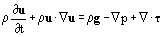where ρ denotes the density of the fluid, u velocity, p pressure, τ deviatoric stress, g gravitational acceleration and t time. Note that it is assumed that τ is a symmetrical tensor, thus ensuring that angular momentum is conserved. Determination of the flow of any fluid requires solution of these conservation equations, subject to appropriate boundary and initial conditions, together with constitutive equations for the fluid. It is in the constitutive equations that the nature of a given fluid is manifested. For a Newtonian fluid τ is given by:
(3)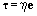where e denotes the strain-rate given by:
(4)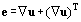and η denotes the viscosity of the fluid, which is independent of e (though it may, of course, depend on temperature T and perhaps on pressure p). A non-Newtonian fluid is one for which Eq. (3) does not hold or one for which Eq. (3) holds but only with η dependent on e.
A flow in a long duct such as a pipe is susceptible to rather simpler analysis than a more general flow because it is essentially a shear flow (which may loosely be regarded as one in which the velocity varies in a direction perpendicular to the direction of flow) as opposed to an extensional flow (which may loosely be regarded as one in which the velocity varies in the direction of flow). In a shear flow, the relevant rheological or flow property is viscosity η and τ is given by Eq. (3). A fluid for which (3) holds but η depends on e is called a generalized Newtonian fluid.
The viscosity η is a scalar; the strain-rate e is a tensor. If η depends on e, then it can be shown [see Richardson (1989) and Bird, Armstrong and Hassager (1977)] that η must be a function of the three scalar invariants Ie, IIe and IIIe of e which are given by:
(5)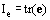(6)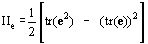(7)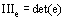where tr( ) and det( ) denote trace and determinant, respectively. For an incompressible fluid:
(8)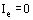A shear flow is one for which:
(9)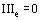Thus IIe is the only nonzero invariant for a shear flow of an incompressible fluid. The shear-rate γ is defined by:
(10)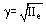Hence the constitutive equation for the stress t of an incompressible generalized Newtonian fluid is:
(11)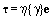For a Newtonian fluid, η is independent of Ie, IIe and IIIe and hence of γ.
Many different semi-empirical relationships for η(γ) exist [(see Bird, Armstrong & Hassager (1977), Skelland (1967) and Wilkinson (1960)]. One of the most widely used is the power-law fluid:
(12)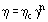where ηc denotes consistency or unit shear-rate viscosity and n the power law exponent. A Newtonian fluid is one for which n = 0. Many non-Newtonian fluids are shear-thinning or pseudoplastic, that is the viscosity h) decreases with increasing shear-rate γ (see Figure 1). The magnitude of the shear stress always increases with increasing shear-rate, however, so the product η γ always increases with increasing γ. Thus −1 < n ≤ 0; typically, n is between −0.8 and 0. If n = 0, the viscosity is, of course, independent of shear-rate; if n = −0.8, an increase in g by a factor of 10 causes a decrease in η by a factor of about 6. For a shear-thickening or dilatant fluid, in contrast, η increases with increasing γ (see Figure 1).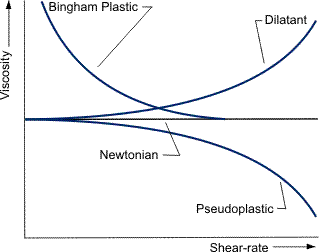Figure 1. Purely viscous fluids.
A relationship which is applicable to fluids which have a yield stress is the Bingham plastic or Bingham fluid:
(13)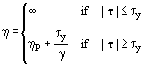(see Figure 1), where ηp denotes the plastic viscosity and τγ the yield stress. If |τ| ≤ τγ, that is the stress in the fluid is less than its yield stress, the fluid does not deform and so γ = 0 but η = ∞ since |τ| ≠ 0 in general. In contrast if |τ| > τγ the fluid deforms and γ is linearly related to |τ|. If |τ| >> τγ then η - ηp. For a Newtonian fluid, τγ = 0.
A somewhat more complicated relationship, which combines features of the power-law fluid and Bingham plastic, so that it is often called a yield power-law fluid, is the Herschel-Bulkley fluid:
(14)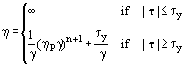which reduces to the Bingham plastic if n = 0 and to the power-law fluid if τy = 0 and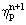is identified with ηc.
A large number of other generalized Newtonian fluids exist [see Bird, Armstrong & Hassager (1977), Skelland (1967), Tanner (1985) and Wilkinson (I960)]. These can, for example, involve the introduction of a characteristic time or a limiting viscosity at high or low shear-rates and hence generally involve further parameters. Thus the Newtonian fluid involves one parameter, η; the power-law fluid involves two, ηc and n; the Bingham plastic involves two, ηp and τy; the Herschel-Bulkley fluid involves three, ηp, τy and n.
In some fluids, the viscosity h varies with time t when the shear-rate g is held constant. Two different types of behavior can be distinguished [see Skelland (1967) and Wilkinson (1960)]. For a thixotropic fluid, η decreases with increasing t; for a rheopectic fluid, η increases with increasing t (see Figure 2). The reason for the variation of η with t is associated with changes in the structure of the fluid. When straining of the fluid breaks down that structure, for example, by destroying local linkages within the fluid, η decreases with increasing t and the fluid is thixotropic. A similar argument can be advanced for the decrease in η with increasing γ for a pseudoplastic fluid. In contrast, when straining builds up the structure, for example by causing local alignment in the fluid, η increases with increasing t and the fluid is rheopectic. Again, a similar argument can be advanced for the increase in η with increasing γ for a dilatant fluid. There is, therefore, an analogy between thixotropy and pseudoplasticity on the one hand and between rheopexy and dilatancy on the other. Nevertheless, variation of viscosity with time is different from, and should not be confused with, variation with shear-rate.Figure 2. Time-dependent fluids.
Constitutive equations for non-Newtonian fluids which are not purely viscous are considerably more difficult to develop and, because of their complexity, are considerably more difficult to use. The constitutive equations in general define what is called the extra stressτE: the deviatoric stress τ in Equation (2) is related to τE by:
(15)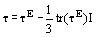where I denotes the unit tensor. There are five main principles which can be used to deduce equations for τE. In a purely mechanical theory (which thus ignores thermal effects and so on) for a purely viscous fluid, the first three of these principles are:
• translational invariance: τE depends on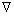u and not on u;
• local action: τE at a given spatial position x depends only on the flow in the neighborhood of x,
• reference-frame indifference or rotational invariance: it can be shown [see Leigh (1968)] that this means that τE depends on e given by (4) and not on vorticity w given by:
(16)It can then be shown [(see Richardson (1989) and Leigh (1968)] that:
(17)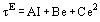where A, B and C are constants depending on Ie, IIe and IIIe given by (5), (6) and (7), respectively. For an incompressible fluid, the term A I can be subsumed into pressure p. Generalized Newtonian fluids are thus fluids for which B = η and C = 0. A fluid for which B = η and C ≠ 0 is called a Reiner-Rivlin fluid. For elasticoviscous fluids, a fourth principle is added:
• determinism: τE for a given material point X at time t depends on the deformation of X at all times t* ≤ t and not t* > t. A typical constitutive equation might then be:
(18)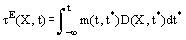where m( ) denotes a memory function and D( ) a deformation measure. An elastic material has a perfect memory in the sense that, however it is deformed, it will always try to revert to its original shape. A viscous material, in contrast, has no memory and never tries to revert to its original shape. An elasticoviscous material has a fading memory; it remembers the recent past better than the distant past. A typical functional form for m( ) is:
(19)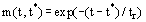where tr denotes a relaxation time. Constitutive equations can be written in integral form, such as Eq. (18), or in differential form. A widely used one in differential form is the Oldroyd fluid:
(20)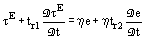where tr1 and tr2 denote relaxation times and:
(21)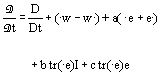where a, b and c are constants. Thus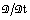is a generalization of the substantial derivative D/Dt given by:
(22)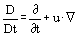D/Dt allows for translation following a given material point X:allows for rotation and stretching as well as translation following X. A special example of the Oldroyd fluid is the Maxwell fluid for which tr2 = 0 and tr1 = tr. For very small deformations, it can be shown that:
(23)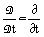and hence that, for a Maxwell fluid:
(24)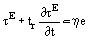For a nearly steady flow, that is one for which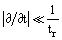:
(25)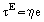which is the constitutive equation for a Newtonian fluid. In contrast for a rapidly changing flow, that is one for which:
(26)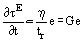where G denotes the shear modulus. Thus Eq. (26) can be integrated to yield:
(27)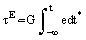But the time-integral of the strain-rate e is just the strain so Eq. (22) is the constitutive equation for a Hookean solid. Thus the Maxwell fluid can be seen to possess both viscous and elastic characteristics.
The study of the flow of non-Newtonian fluids is called rheology: measurements are made in rheometers [see Walters (1975)]. In addition to viscosity, or strictly shear viscosity, η, three other parameters are of interest. They are extensional viscosity ηextfirst normal stress difference coefficient Ψ1 and second normal stress difference coefficient Ψ2. This is because, in addition to Shear Stress which is normally important for Newtonian fluids, Normal Stress is also important for non-Newtonian fluids. For a simple shear flow (see Figure 3):
(28)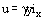where ix denotes a unit vector in the x-direction. The stress τ comprises nine components. For a Newtonian fluid, seven of these (τxz, τzx, τyz, τzy, τxx, τyy and τZZ) vanish for a simple shear flow. For a non-Newtonian fluid in contrast, only four of these (τxz, τzx, τyz and τzy) vanish. Of those which do not vanish:
(29)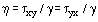(30)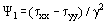(31)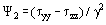For a pseudoplastic fluid such as a molten polymer, η is positive and decreases as γ increases; Ψ1 is positive and decreases as γ increases and Ψ2 is negative and increases as γ increases (see Figure 4).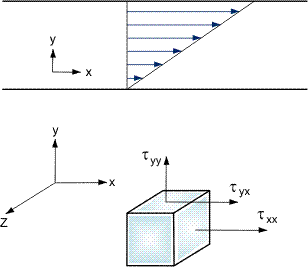Figure 3. Simple shear flow.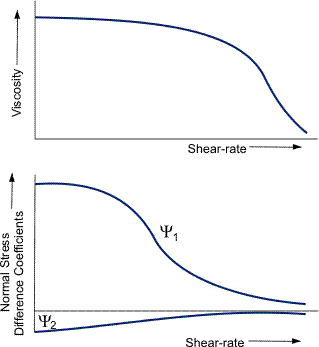Figure 4. Pseudoplastic fluids.
For a simple extensional flow with extension-rate e (see Figure 5):
(32)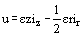where iz and ir denote unit vectors in the z-direction and r-direction, respectively. By definition:
(33)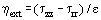For a Newtonian fluid:
(34)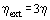For a pseudoplastic fluid, ηext is greater than 3 η and increases as ε increases: ηext can be several orders of magnitude larger than η at high ε.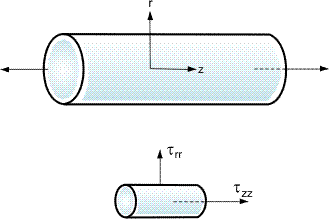Figure 5. Simple, extensional flow.
Non-Newtonian fluids exhibit a number of effects not shown by Newtonian fluids. A Newtonian fluid issuing at high Reynolds number from an orifice of diameter D will tend to form a jet of diameter 0.87D, whereas a non-Newtonian fluid issuing in the same way, though normally at low Reynolds number because of the higher viscosity involved, will tend to form a jet of typical diameter 3D. This so-called extrudate swell has important consequences, for example, in the formation of droplets. Rod-climbing, as the name suggests, means that a non-Newtonian fluid will, because of normal stress effects, tend to climb up a rotating rod, whereas a Newtonian fluid will tend to move down the rod because of centrifugal effects. This has important consequences, for example, in the stirring of reactors. Drag-reduction is the significant reduction in friction factor, typically by two orders of magnitude, for turbulent flow of certain non-Newtonian fluids such as polymer solutions in water compared with that of the corresponding Newtonian fluid such as water. Unfortunately, the reduction in friction factor is accompanied by a similar reduction in heat transfer coefficient, so there is no obvious application of this effect to flows in heal exchangers.